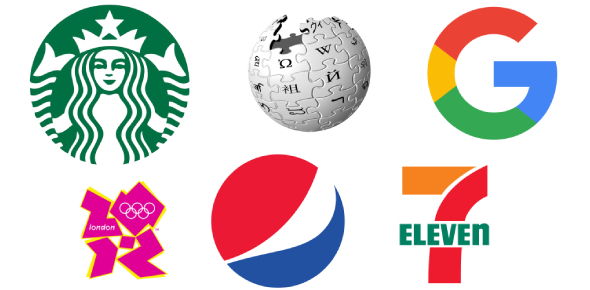Unit 3 Target 3: pH Quiz

8 Questions | Total Attempts: 768Settings• 1.
Which solution has the greatest concentration of hydroxide ions (OH-)?
• A.

Urine (pH 6.0)

• B.

Rainwater (pH 5.5)

• C.

Tomato juice (pH 4.0)

• D.

Gastric juice (pH 2.0)

• 2.
When the pH in a stomach increases from 2 to 4, how does the hydrogen ion concentration change?
• A.

It increases by a factor of 2.

• B.

It increases by a factor of 100.

• C.

It decreases by a factor of 2.

• D.

It decreases by a factor of 100.

• 3.
As part of an experiment, a student adds 10 mL of 5% hydrochloric acid solution to 100 mL of a non-buffered, colorless solution of sugar.  What is the most likely result?
• A.

The concentration of hydroxide ions (OH-) will increase.

• B.

The concentration of hydrogen ions (H+) will increase.

• C.

The pH will not change.

• D.

The pH will increase.

• 4.
Netural solutions have a pH of
• A.

0

• B.

7

• C.

9

• D.

14

• 5.
Which is true?
• A.

PH of less than 7 is basic; pH of more than 7 is acidic

• B.

PH of less than 7 is acidic; pH of more than 7 is basic

• C.

Option 3

• D.

Option 4

• 6.
If a solution has 455,000 H+ at at pH of 3, how many H+ would there be at a pH of 5?
• A.

455

• B.

4,550

• C.

45,500,000

• D.

4,550,000

• E.

45,500

• 7.
If a solution has 9,700 H+ at a pH of 8, how many H+ would there be at a pH of 5?
• A.

970

• B.

9.70

• C.

97,000

• D.

970,000

• E.

9,700,000

• 8.
If a solution has 420,000 OH- at a pH of 8, how many OH- would there be at a pH of 9?
• A.

4,200

• B.

42,000,000

• C.

4,200,000

• D.

42,000

• E.

420

Related TopicsBack to top